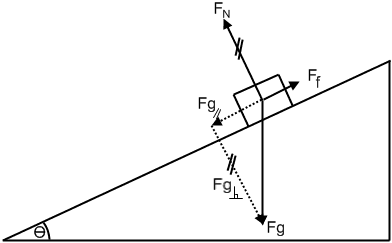# Coefficient of friction on an inclined plane (1 Viewer)

### Users Who Are Viewing This Thread (Users: 0, Guests: 1)

#### Will M

[SOLVED] Coefficient of friction on an inclined plane

1. The problem statement, all variables and given/known data
The problem I have is to determine the coefficient of kinetic friction between two wooden objects, a block and a ramp, using the fewest measurements possible. The initial velocity will be 0. I can measure the angle of incline, the distance travelled along the ramp, the time taken, but should not have to measure mass. I do not currently have any values, but should create an equation. Can I do this, and if so, am I on the right track?

2. Relevant equations
Fnet = ma
Ff = μFN
Δd = V1Δt + ½aΔt²

3. The attempt at a solutionFnet = ma

Ff - Fg║ = ma

Ff - Fg sin θ = ma

Ff - mg sin θ = ma

Ff = 2Δd/Δt² - g sin θ

μFN = 2Δd/Δt² - g sin θ

μFg┴ = 2Δd/Δt² - g sin θ

μFg cos θ = 2Δd/Δt² - g sin θ

μ = [(2Δd/Δt²) - g sin θ] / Fg cos θ

μ = [(2Δd/Δt²) - g sin θ] / mg cos θ

Note:The substitution of acceleration for 2Δd/Δt² in the 5th line is from the equation Δd = V1Δt + ½aΔt² where V1Δt evaluates to 0.

#### Snazzy

Shouldn't it be Fg - Ff = ma since Fg is the leading force causing the acceleration and Ff is the retarding force?

Also, you can express Fn in simpler terms to cancel out the masses.

#### Will M

How would I express FN in simpler terms?

#### Snazzy

What component of the weight is opposing the normal force? You can express all the Fg components in terms of m, and then cancel out m.

#### Will M

Ok, I realize that since μ is a constant, it shouldn't be mass dependent. I also know that since there is no acceleration in the perpendicular direction, the normal force is opposed by, and has a magnitude equal to, the perpendicular component of the force of gravity. I've expanded this to mg cos θ but I don't have another m to cancel the mass out with.
Could you please point out the line where I've gone wrong, and what I should have done? Or am I just missing something that I should be doing after the last line?

Sorry for being clueless.#### Snazzy

You know that the block has a mass m. Therefore, your applied force is mgsinX. You can figure out what the normal force is equal to in terms of mg with your free body diagram and then use this to show what the frictional force is equal to.

You also know that Fnet = ma.

Thus you can cancel mass and substitute a kinematics equation to find the distance.

#### Will M

There is no applied force, do you mean net force? Also, I'm looking for the coefficient of friction, not the force of friction, otherwise I would have stopped at the line Ff = 2Δd/Δt² - g sin θ

#### Snazzy

Right, there is no applied force. I meant the force that is causing the acceleration, namely the F_g (parallel) force.

You know that $$F_g_/_/=mgsin\theta$$

You know that $$F_f=\mu F_n$$

But what is Fn? How can you express this in terms of m?

#### Will M

I could express it as $$F_N=mgcos\theta$$ right?

Then I could substitute it for $$F_N$$ in $$F_f=\mu F_n$$

After rearranging to solve for μ, it would be $$\mu=F_f/mgcos\theta$$

Substituting the fifth line of my original solution for $$F_f$$ would give me

$$\mu = \left(2\Delta d/\Delta t^{2} - g sin \theta\right)/mgcos\theta$$

Which is where I get confused. I can't think of any other way to express $$F_N$$

#### Snazzy

$$mgcos\theta - F_f = ma$$

$$mgcos\theta - \mu F_n = ma$$

$$mgcos\theta - \mu mgsin\theta=ma$$

Can you solve it now?

#### Will M

Ah, I think I see it now. Thanks!Would this be correct:

$$mgsin\theta - \mu mgcos\theta=ma$$

$$mg(sin\theta - \mu cos\theta)=ma$$

$$g(sin\theta - \mu cos\theta)=a$$

$$\mu g +g(sin\theta - cos\theta)=a$$

$$\mu +sin\theta - cos\theta=a/g$$

$$\mu=a/g -sin\theta + cos\theta$$

$$\mu=2\Delta d/\Delta t^{2}g -sin\theta + cos\theta$$

#### Snazzy

Your algebra is off starting with the fourth step.

#### Will M

Yeah, I caught that when I checked it over, but thanks, because you've been a real help!### The Physics Forums Way

We Value Quality
• Topics based on mainstream science
• Proper English grammar and spelling
We Value Civility
• Positive and compassionate attitudes
• Patience while debating
We Value Productivity
• Disciplined to remain on-topic
• Recognition of own weaknesses
• Solo and co-op problem solving